Wir verwenden Google für unsere Suche. Mit Klick auf „Suche aktivieren“ aktivieren Sie das Suchfeld und akzeptieren die Nutzungsbedingungen.

# New in SG-Lib 2.4

###### SGweight(SG,sw,res)- returns the estimated weight of a solid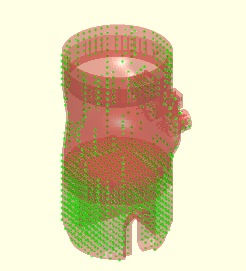% SGweight(SG,sw,res) - returns the estimated weight of a solid% (by Tim Lueth, VLFL-Lib, 2015-AUG-26 as class: MECHANICAL PROCEDURES)% % Calculated by counting the volume elements that are included by the % solid body. (Status of: 2015-08-27)% % See also: SGweight, SGvolume% % W=SGweight(SG,[sw,res])% === INPUT PARAMETERS ===% SG: Solid Geoemtry% sw: specific weight; default is 1.15g/cm^3 (Nylon)% res: resolution; default is 2x2x2 mm^3% === OUTPUT RESULTS ======% W: Estimated weight in gramm%
###### TofSGMLez(SG,ez,ai,fe)- returns HT for a mounting face with known ez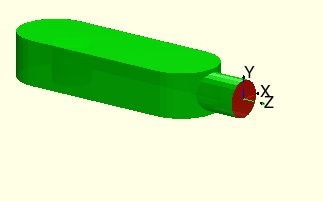% TofSGMLez(SG,ez,ai,fe) - returns HT for a mounting face with known ez% (by Tim Lueth, VLFL-Lib, 2015-AUG-24 as class: ANALYTICAL GEOMETRY)% % % Similar to TofSGML but here, the faces are seleted by the ez-vector % (Status of: 2017-01-29)% % See also: TofSGMLez, TofSGML, TofVLUL, TofVLULez, MLofSG, MLplot% % T=TofSGMLez(SG,ez,[ai,fe])% === INPUT PARAMETERS ===% SG: Solid Geometry with defined mounting faces ML, MA% ez: desired ez vector% ai: order is size; default is 1% fe: feature edge angle; default is 0.5; used only if ML is not defined% === OUTPUT RESULTS ======% T: Transformation matrix [4x4]% % EXAMPLE: % load AIM_SGrobot% TofSGMLez(SG3,[1 0 0]);%
###### TofSGML(SG,mi,fe)- returns HT for a mounting face with known number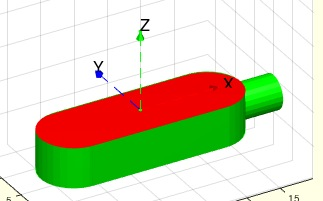% TofSGML(SG,mi,fe) - returns HT for a mounting face with known number% (by Tim Lueth, VLFL-Lib, 2015-AUG-24 as class: ANALYTICAL GEOMETRY)% % % Similar to TofSGMLez but here, the faces are seleted by the number % (Status of: 2017-01-29)% % See also: TofSGMLez, TofVLUL, TofVLULez, MLofSG, MLplot% % [T,SG]=TofSGML(SG,mi,[fe])% === INPUT PARAMETERS ===% SG: Solid Geometry with defined mounting faces ML, MA% mi: mounting face number% fe: feature edge angle; default is 0.5; used only if ML is not defined% === OUTPUT RESULTS ======% T: Transformation matrix [4x4]% SG: Solid geometry with defined mounting faces ML, MA% % EXAMPLE: % load AIM_SGrobot% TofSGML(SG3,2);%
###### MLplot(SG,mi,fe)- plots the mounting faces of a solid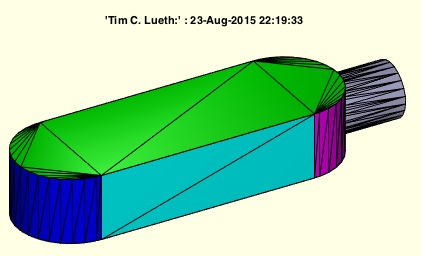% MLplot(SG,mi,fe) - plots the mounting faces of a solid% (by Tim Lueth, VLFL-Lib, 2015-AUG-23 as class: SURFACES)% % calculates using MFofSG, if not existing already, the mounting faces % and plots them in different colors (Status of: 2017-01-29)% % See also: TofSGMLez, TofSGML, TofSGMLez, MLofSG, SGplatesofSGML% % h=MLplot(SG,[mi,fe])% === INPUT PARAMETERS ===% SG: Solid Geoemtry% mi: mounting face index; default is ''% fe: feature angle; default is 0.5% === OUTPUT RESULTS ======% h: handle% % EXAMPLE: % [~,~,SG]=MLofSG(SGsample(5)); MLplot(SG)%
###### SGimpress (A,B,TA,TB,ai,bi)- impresses solid B into A using mounting faces% SGimpress (A,B,TA,TB,ai,bi) - impresses solid B into A using mounting % faces% (by Tim Lueth, VLFL-Lib, 2015-AUG-23 as class: SURFACES)% % SGimpress([A,B,TA,TB,ai,bi])% === INPUT PARAMETERS ===% A: Solid A% B: Solid B% TA: Vector or HT-Matrix of A% % TB: Vector or HT-Matrix of B% ai: order index of mounting face of A; default 1 (biggest of this ez)% bi: order index of mounting face of B; default 1 (biggest of this ez)%
###### rotateazimut(hf)- callback func for figure-azimut-rotating by wheel% rotateazimut(hf) - callback func for figure-azimut-rotating by wheel% (by Tim Lueth, VLFL-Lib, 2015-AUG-23 as class: USER INTERFACE)% % The procedure can be called as part of set procedure:% set (hf,'WindowScrollWheelFcn',@figrotateazimut);% (Status of: 2015-08-23)% % rotateazimut(hf)% === INPUT PARAMETERS ===% hf: handle to figure% % EXAMPLE: set (hf,'WindowScrollWheelFcn',@figrotateazimut);%
###### exp_2015_08_22- EXPERIMENT to show simple impression of objects in planes%% PUBLISHABLE EXP_2015_08_22 EXPERIMENT TO SHOW SIMPLE IMPRESSION OF OBJECTS IN PLANES% (by Tim Lueth, VLFL-Lib, 2015-AUG-22 as class: EXPERIMENTS)%%% exp_2015_08_22 - EXPERIMENT to show simple impression of objects in % planes% (by Tim Lueth, VLFL-Lib, 2015-AUG-22 as class: EXPERIMENTS)% % exp_2015_08_22%
###### tangentRP(R,p,pc)- returns the two tangential points of a circle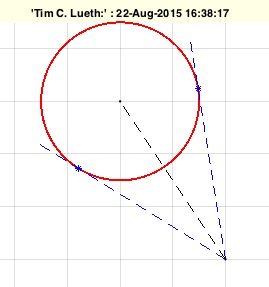% tangentRP(R,p,pc) - returns the two tangential points of a circle % (by MATLAB-CENTRAL, VLFL-Lib, 2015-AUG-22 as class: ANALYTICAL GEOMETRY)% % Thanks to Laurens de Smedt for finding it at MATLAB-Central% (Status of: 2017-01-31)% % See also: center3P, center4P, tangente, tangenteRP% % [q1,q2]=tangentRP(R,p,[pc])% === INPUT PARAMETERS ===% R: Radius of the circle% p: point to match% pc: optional center of the circle; default is [0 0]% === OUTPUT RESULTS ======% q1: point 1% q2: point 1% % EXAMPLE: tangentRP(10,[20 20])%
###### PLFLofVLELdelaunay(VL,EL)- closes a planar surface of VLEL by delaunay% PLFLofVLELdelaunay(VL,EL) - closes a planar surface of VLEL by delaunay% (by Tim Lueth, VLFL-Lib, 2015-AUG-21 as class: SURFACES)% % This procedure can be called also just as FLofVLELdelaunay(VL,EL) % (Status of: 2015-08-21)% % [PL,FL,EL]=PLFLofVLELdelaunay(VL,EL)% === INPUT PARAMETERS ===% VL: Vertex list [nx3]% EL: Edge consraints% === OUTPUT RESULTS ======% PL: Point List% FL: Tesselated facet list% EL: Same Edge List%
###### PLofVLFL(VL,FL,fi)- returns the 2D representation for a planar VLFL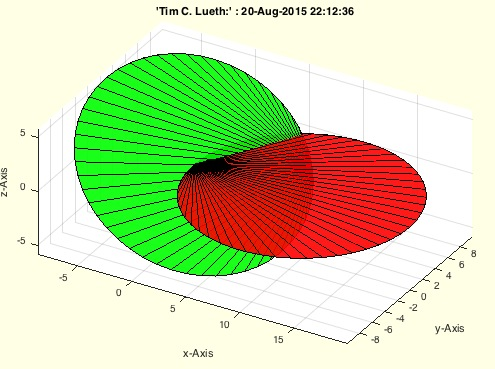% PLofVLFL(VL,FL,fi) - returns the 2D representation for a planar VLFL % (by Tim Lueth, VLFL-Lib, 2015-AUG-20 as class: SURFACES)% % This procedure analyzes generates a HT-Matrix by the first point of the % first facet, the ez-vector of the surface, and the longest distance to % another point of the facets as ex-axis. (Status of: 2015-08-20)% % [PL,T,c,d]=PLofVLFL(VL,FL,[fi])% === INPUT PARAMETERS ===% VL: Vertex list [nx3]% FL: Facet list of a planar surface [nx3]% fi: selected indices; default is all% === OUTPUT RESULTS ======% PL: Point list [nx2]% T: Transformation matrix% c: index list to transform PL indices into VL indices% d: index list to transform VL indices into PL indices% % EXAMPLE: FL=FLofn(50);% VL=VLtrans(PLcircle(10,50),rotdeg(20,30,40));% PLofVLFL(VL,FL(30:40,:)); % [PL,T,c,d]=PLofVLFL(VL,FL(30:40,:)); c, d(FL(30:40,:))%
###### vertexNormalbyFN(TR3)- calculates the vertex normals based on the facet normals% vertexNormalbyFN(TR3) - calculates the vertex normals based on the facet normals% (by Tim Lueth, VLFL-Lib, 2015-AUG-21 as class: SURFACES)% % This fnctn is sometimes required to improve the result of MATLAB's % vertexNormal fnctn. It is about 40 times slower (Status of: 2017-07-08)% % Introduced first in SolidGeometry 2.4% % See also: vertexNormal% % [NNL,NL]=vertexNormalbyFN(TR3)% === INPUT PARAMETERS ===% TR3: triangulation% === OUTPUT RESULTS ======% NNL: Normal vector list% NL: vector sum of the face norms attached to the vertex% % EXAMPLE: % vertexNormalbyFN(SGbox([30,20,10]))% vertexNormalbyFN(SGsample(25))% % See also: vertexNormal%
###### incenterVLFL(VL,FL)- returns the center of a surface and of all facets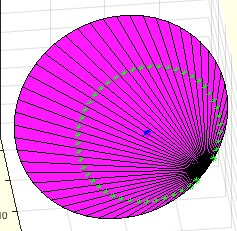% incenterVLFL(VL,FL) - returns the center of a surface and of all facets% (by Tim Lueth, VLFL-Lib, 2015-AUG-21 as class: SURFACES)% % [CVL,SCV]=incenterVLFL(VL,FL)% === INPUT PARAMETERS ===% VL: Vertex list [nx2] or [nx3]% FL: Facet list% === OUTPUT RESULTS ======% CVL: Center Vertex List% SCV: Surface Center Vertex%
###### PLofVL(VL)- Converts a planer VL[nx3] into a PL [nx2]% PLofVL(VL) - Converts a planer VL[nx3] into a PL [nx2]% (by Tim Lueth, VLFL-Lib, 2015-AUG-21 as class: AUXILIARY PROCEDURES)% % Required only for procedure parameters (Status of: 2015-08-21)% % [PL,T]=PLofVL(VL)% === INPUT PARAMETERS ===% VL: Vertex list [nx3]% === OUTPUT RESULTS ======% PL: Point list [nx2]% T: Transformation matrix for transformation PL->VL% % EXAMPLE: % PLofVL(VLtrans(PLcircle(10,13,[],8),rotdeg(90,90,90)));%
###### exp_2015_08_20b (SG,k)- EXPERIMENT just for testing retesselation by ULofSG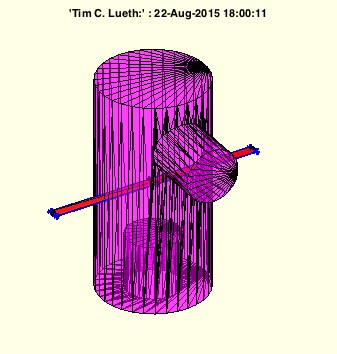% exp_2015_08_20b (SG,k) - EXPERIMENT just for testing retesselation by % ULofSG% (by Tim Lueth, VLFL-Lib, 2015-AUG-20 as class: EXPERIMENTS)% % exp_2015_08_20b(SG,k)% === INPUT PARAMETERS ===% SG: Solid Geometry% k: index for surface retesselation% % EXAMPLE: load AIM_SGrobot% exp_2015_08_20c(SG1)%
###### exp_2015_08_20c(SG,k)- EXPERIMENT just for retesselation by ULofSG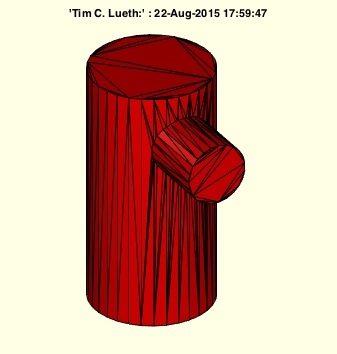% exp_2015_08_20c(SG,k) - EXPERIMENT just for retesselation by ULofSG% (by Tim Lueth, VLFL-Lib, 2015-AUG-20 as class: EXPERIMENTS)% % NSG=exp_2015_08_20c(SG,k)% === INPUT PARAMETERS ===% SG: Solid Geometry% k: index for surface retesselation% === OUTPUT RESULTS ======% NSG: New Solid% % EXAMPLE: load AIM_SGrobot% exp_2015_08_20c(SG1)%
###### SGshort(SG,thr,sil)- returns a new SG with may be shorter VL and FL% SGshort(SG,thr,sil) - returns a new SG with may be shorter VL and FL% (by Tim Lueth, VLFL-Lib, 2015-AUG-20 as class: SURFACES)% % Similar to VLFLselect or VLELselect. The fnctn makes it impossible to % separate facets later. Be careful when using it. Do not use it if you % want to modify the VL or FL manually later. It is an optimization fnctn % used in SGgrow.% using the input argument 'sil', it is possible to hide the message on % removed vertices (Status of: 2017-02-13)% % [SG,n]=SGshort(SG,[thr,sil])% === INPUT PARAMETERS ===% SG: Solid Geoemtry% thr: threshold for vertex fusion; default is 1e-12% sil: silence; default is false% === OUTPUT RESULTS ======% SG: SG% n: doubled an removed vertices%
###### SGcontourtube(CPL,VL,VLO)- extrudes a CPL along a path given as CVL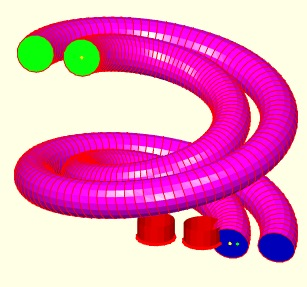% SGcontourtube(CPL,VL,VLO) - extrudes a CPL along a path given as CVL% (by Tim Lueth, VLFL-Lib, 2015-AUG-19 as class: SURFACES)% % =======================================================================% OBSOLETE (2017-07-09) - USE FAMILY 'SGofCPLCVLR' INSTEAD% =======================================================================% % Powerful procedure to extrude PLs [nx2] anlog a path (Status of: % 2017-07-09)% % Introduced first in SolidGeometry 2.4% % See also: [ SGofCPLCVLR ] ; SGofCPLCVLR, RLofEulerInterpolation, % VLinsertEulerSteps, VLradialEdges, TofPez% % [SG,FLS,FLE,FLW,TS,TE]=SGcontourtube(CPL,VL,VLO)% === INPUT PARAMETERS ===% CPL: Closed point list [nx2]% VL: Vertex list [nx3]% VLO: Second vertex list [nx3]% === OUTPUT RESULTS ======% SG: Solid geometry% FLS: FL of starting surface% FLE: FL of ending surface% FLW: FL of the wall% TS: Starting Frame% TE: End Frame% % EXAMPLE: % VL=VLhelix(40,100,3*pi); VLO=[VL(:,1:2)*1.1 VL(:,3)];% SGcontourtube(PLcircle(10),VL,VLO)% % See also: [ SGofCPLCVLR ] ; SGofCPLCVLR, RLofEulerInterpolation, % VLinsertEulerSteps, VLradialEdges, TofPez%
###### VLtransN(VLO,FLO,s,d)- returns a relative to normal vectors transformed vertex list% VLtransN(VLO,FLO,s,d) - returns a relative to normal vectors transformed vertex list% (by Tim Lueth, VLFL-Lib, 2015-AUG-19 as class: SURFACES)% % See also: VLtransT, VLtransP, VLtrans1, VLtrans0, VLtransR, VLtransT, % VLtrans% % [VL,EL,EOL,FL,VNL]=VLtransN(VLO,FLO,[s,d])% === INPUT PARAMETERS ===% VLO: Vertex list of a planar surface% FLO: Facet list of a planar surface% s: shrink factor% d: distance factor% === OUTPUT RESULTS ======% VL: Vertex list [nx3]% EL: Edge list [nx2]% EOL: Edge orthogonal list [nx3]% FL: Facet list [nx3]% VNL: Vertex normal list [nx3]%
###### exp_2015_08_20 (SG)- EXPERIMENT to connect two surfaces by a small tube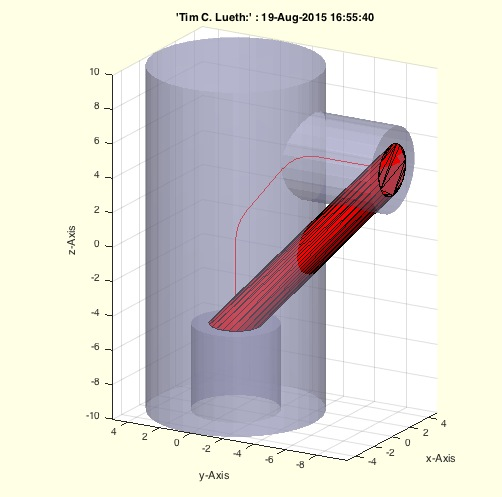% exp_2015_08_20 (SG) - EXPERIMENT to connect two surfaces by a small tube% (by Tim Lueth, VLFL-Lib, 2015-AUG-19 as class: EXPERIMENTS)% % exp_2015_08_20(SG)% === INPUT PARAMETERS ===% SG: % % EXAMPLE: load AIM_SGrobot% exp_2015_08_20(SG1)%
###### MLofSG(SG,fi,fe,amin)- calculates of the mounting face list of the solid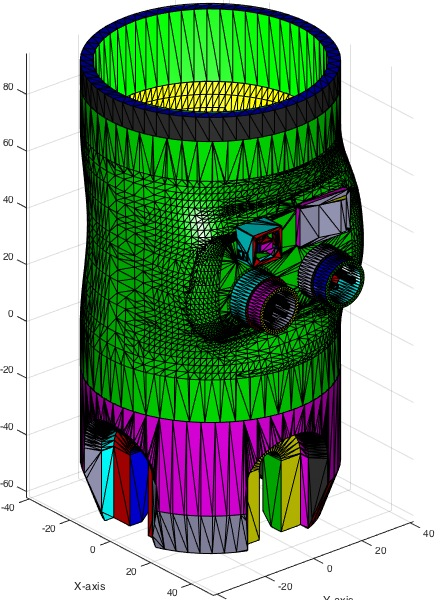% MLofSG(SG,fi,fe,amin) - calculates of the mounting face list of the solid% (by Tim Lueth, VLFL-Lib, 2015-AUG-19 as class: SURFACES)% % MLofSG uses TR3mountingfaces to calculate the desired union faces. % Therefore, it is able to handel spherical faces too. If only planar % faces are allowed (i.e. fe=0) , the fnctn is up to 10 to 25 times % faster. The slower plotting if nargout=0 by drawnow slows down but % allows user interruption!% ML contains for all faces of FL, the index of the mounting face% The first column of MA contains the mounting face index Ai(:,1)% The second column of MA contains the area of the union surface Ai(:,2)% the 3rd-5th column of MA contains the normal vector length Ai(:,3:5).% The normal vector was calculated by the sum of all normal vectors % divided by the number of faces, therefor for spherical mounting faces, % the norm of Ai(i, 3:5) is not 1 but smaller. Use % [a,b]=VLnorm(MA(:,3:5)) to find the non planar surfaces. In this case b % is smaller than 0. % (Status of: 2017-01-29)% % See also: TofSGMLez, TofSGML, TofSGMLez, MLplot, SGplatesofSGML% % [ML,MA,SG]=MLofSG(SG,[fi,fe,amin])% === INPUT PARAMETERS ===% SG: Solid Geometry% fi: facet index or empty% fe: feature edge angle (0.3) is default% amin: minimal facet are to consider as start point;default is 0% === OUTPUT RESULTS ======% ML: Mounting face index list of FL (nx1), n=length(FL)% MA: Array [nx5]= [mounting area index(1) area (1) normal vector(3)]% SG: SG.ML und SG.MA added using ML and MA if fi=[];% % EXAMPLE: load AIM_SGrobot; % SG=SG1, fe=0.5, MLofSG(SG,[],fe);% [ML,MA]=MLofSG(SG,[],fe), MA(:,[1 3 4 5]), [a,b]=VLnorm(MA(:,3:5))% SG.FL(ML==4,:), % return the facets that belong to union 4% SG.FL(ML~=4,:), % return the facets that do not belong to union 4%
###### OcTree(pts,)-###### exp_2015_08_19(A,B,oct)- EXPERIMENT that shows an octree separation% exp_2015_08_19(A,B,oct) - EXPERIMENT that shows an octree separation% (by Tim Lueth, VLFL-Lib, 2015-AUG-19 as class: EXPERIMENTS)% % exp_2015_08_19(A,B,[oct])% === INPUT PARAMETERS ===% A: Solid A% B: Solid B% oct: octree size ([oct x oct x oct]); default is 40% % EXAMPLE: % exp_2015_08_19([],[]) %
###### octMesh(facesA,pointsA,facesB,pointsB,octs)- Splits two triangular meshes into a single system of octree spatial bins###### CPLunite(CPL,ord)- returns a CPL that has no crossing lines anymore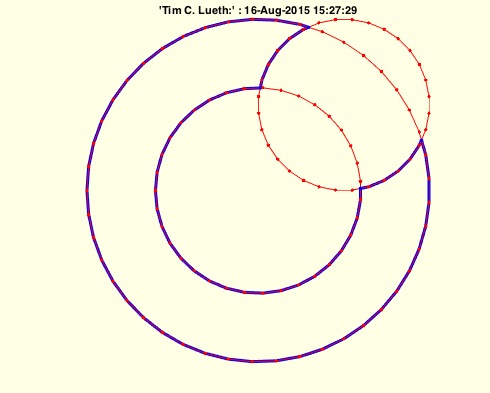% CPLunite(CPL,ord) - returns a CPL that has no crossing lines anymore% (by Tim Lueth, VLFL-Lib, 2015-AUG-16 as class: CLOSED POLYGON LISTS)% % By analyzing the order (clockwise or counterclockwise) of all polygons % a addition or substraction is executed. Therefor the final CPL has no % crossings anymore.% ATTENTION: THERE IS A DIFFERENT CPLunitCPL that does not the same % (Status of: 2015-08-16)% % See also: CPLunite, CPLuniteCPL, CPLunitePLFL% % CPLA=CPLunite(CPL,[ord])% === INPUT PARAMETERS ===% CPL: CPL including crossing lines% ord: true if CPLorder is used first; default is false% === OUTPUT RESULTS ======% CPLA: Processed CPL% % EXAMPLE: % CPLunite([PLcircle(10);NaN NaN;flipud(PLtrans(PLcircle(5),[5 5]))]);% CPLunite([PLcircle(10);NaN NaN;PLtrans(PLcircle(5),[5 5])]);% CPLunite([flipud(PLcircle(6));NaN NaN;PLcircle(10);NaN % NaN;flipud(PLtrans(PLcircle(5),[5 5]))])% CPLunite([flipud(PLcircle(6));NaN NaN;PLcircle(10);NaN % NaN;PLtrans(PLcircle(5),[5 5])])%
###### VLELofTR(TR)- returns the edge constraints of a triangulation% VLELofTR(TR) - returns the edge constraints of a triangulation% (by Tim Lueth, VLFL-Lib, 2015-AUG-15 as class: TETRAHEDRONS)% % returns the edge constraints of a triangulation (Status of: 2015-08-15)% % [VL,EL,FL]=VLELofTR(TR)% === INPUT PARAMETERS ===% TR: Triangulation (nx4 or nx3 or nx2)% === OUTPUT RESULTS ======% VL: Vertex List (Point List)% EL: Facet List (Constraints)% FL: Facet List (ConnectivityList or freeBoundary)% % EXAMPLE: % [VL,FL]=VLELofTR(exp_2014_12_23 (SGsample(16))); close all; VLFLfigure; % view(-30,30); VLFLplots(VL,FL);%
###### exp_2015_08_09- experiment to show how to create kinematic chains by cicking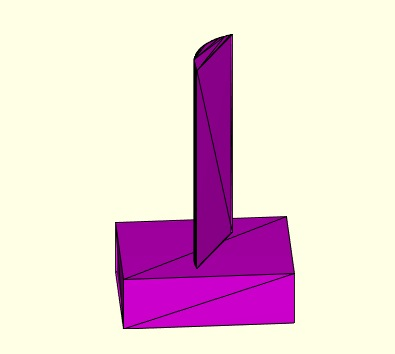% exp_2015_08_09 - experiment to show how to create kinematic chains by % cicking% (by Tim Lueth, VLFL-Lib, 2015-AUG-09 as class: EXPERIMENTS)% % exp_2015_08_09%
###### KMofSGs(SGs,dphi,thr)- returns a Kinematic model for a chain of solids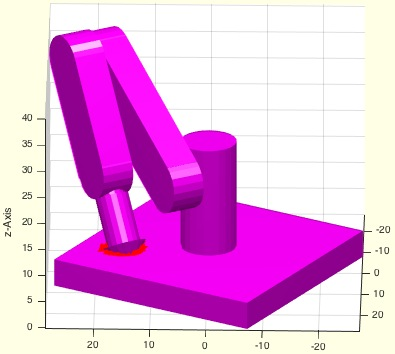% KMofSGs(SGs,dphi,thr) - returns a Kinematic model for a chain of solids% (by Tim Lueth, VLFL-Lib, 2015-AUG-09 as class: KINEMATICS AND FRAMES)% % The solids are given as cell list: {SG1,SG2,SG3...}% The procedure also performs a collision test to give a feedback on the % geometry. For this purpose all facets of the analyzed links are % shrinked by thr=0.1. and compared with all surfaces of the links in the % chain before.% % % At a later stage, it is absolutely necessary to integrate the chains % into the model% KM.m=models (i.e. current KM)% KM.c=chains% (Status of: 2015-08-09)% % [KM,chain]=KMofSGs(SGs,[dphi,thr])% === INPUT PARAMETERS ===% SGs: Cell list of solids: {SG1,SG2,SG3...}% dphi: angle degree (default is 105 degree) for all joints% thr: threshold for collision test; default is 0.1 (==2*0.05)% === OUTPUT RESULTS ======% KM: Kinematic Model including a simple chain% chain: generated chain of frames% % EXAMPLE: Create a strange robot% KMofSGs ({SG0,SG1,SG2,SG2,SG2,SG3,SG2,SG4});%
###### SGfixerrors(A,thr)- removes typical errors after surface manipulation% SGfixerrors(A,thr) - removes typical errors after surface manipulation% (by Tim Lueth, VLFL-Lib, 2015-AUG-08 as class: SURFACES)% % Even after the use of matlab triangulation procedures, there are % sometimes facets that are of edge type. Those errors are removed with % this procedure.% Used in exp_2015_08_08 (Status of: 2015-08-08)% % A=SGfixerrors(A,[thr])% === INPUT PARAMETERS ===% A: Solid A% thr: threshold for VLFLshort2; default is 1e-20;% === OUTPUT RESULTS ======% A: Solid with removed errors%
###### exp_2015_08_08- PUBLISHABLE EXPERIMENT showing the creation of boolean operations%% exp_2015_08_08 - PUBLISHABLE EXPERIMENT showing the creation of boolean operations% (by Tim Lueth, VLFL-Lib, 2015-AUG-08 as class: EXPERIMENTS)%% % exp_2015_08_08%
###### SGofVLtrans(VL,T,TS,comp)- returns a convex solid for a transformed vertex list% SGofVLtrans(VL,T,TS,comp) - returns a convex solid for a transformed % vertex list% (by Tim Lueth, VLFL-Lib, 2015-AUG-08 as class: SURFACES)% % Useful state of the art procedure to move/rotate a base contour first, % the create a convex delaunay solid, a returns it with index lists for % the individual surfaces (uses SGofVLdelaunay).% Translation support: dz, t-vector, 3x3 matrix, 3x4, 4x4 matrices % (Status of: 2015-08-08)% % [SG,ViL,FiL,FNL,FoL]=SGofVLtrans(VL,T,[TS,comp])% === INPUT PARAMETERS ===% VL: Vertex list (nx3) or (nx2)% T: required translation; if scalar the t==>t=[0 0 t];% TS: transformation of the solid afterwards; default is [];% comp: compensates the contraction for phi-x or phi-y; default is false% === OUTPUT RESULTS ======% SG: Solid geoemtry% ViL: Vertex index list for the surface% FiL: Facet index list for the base and tranformed contour% FNL: facet normal list for the base and transformed contour% FoL: Facet index list for the outside shell of the solid% % EXAMPLE: % SGofVLtrans(PLcircle(10,4),[rotdeg(0,0,45),[0 ;0 ;40]]) % rotz-45 and % t=40% SGofVLtrans(PLcircle(10,4),-30); % z=-30%
###### VLtrans(VLA,T)- return similar to PLtrans or SGtrans a transformed VL% VLtrans(VLA,T) - return similar to PLtrans or SGtrans a transformed VL% (by Tim Lueth, VLFL-Lib, 2015-AUG-08 as class: AUXILIARY PROCEDURES)% % Usage of T% 0 Center around origin% 1 Only 1st quadrant% [nx3] Translation vector% [3x3] Rotation matrix% [3x4] Rotation plus translation matrix% [4x4] Fully homogenous transformation matrix (Status of: 2017-01-29)% % See also: VLtransT, VLtransP, VLtrans1, VLtrans0, VLtransR, VLtransT, % VLtransN% % VL=VLtrans(VLA,T)% === INPUT PARAMETERS ===% VLA: Vertex list% T: Transformation vector% === OUTPUT RESULTS ======% VL: tranformed vertex list% % EXAMPLE: close all; SGfigure; view(-30,30)% VLtrans(VLaddz(PLcircle(10,8)),[0 0 40])%
###### VLcrossingSG(SGA,SGB)- returns crossing points of two solid geometries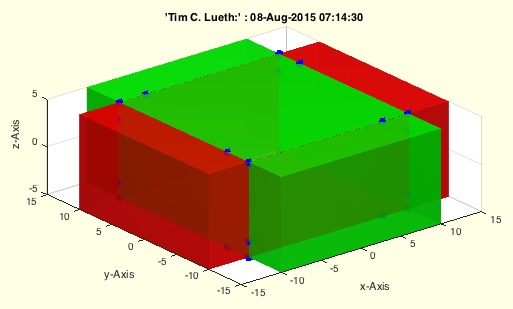% VLcrossingSG(SGA,SGB) - returns crossing points of two solid geometries% (by MATLAB-CENTRAL, VLFL-Lib, 2015-AUG-08 as class: SURFACES)% % Extreme fast, powerful, and useful fnctn % Supports also cell list of Solids (Status of: 2017-01-02)% % See also: mesh2mesh, VLFLinpolyhedron, BBiscollofVL, outboundingbox, % SGisInterior, crossingfacets2VLFL% % VL=VLcrossingSG(SGA,SGB)% === INPUT PARAMETERS ===% SGA: Solid A or cell list of solids% SGB: Solid B or cell list of solids% === OUTPUT RESULTS ======% VL: Vertex list of all crossing point% % EXAMPLE: Crossing points of 2 boxes% A=SGbox([30,20,10]); % VLcrossingSG(A,SGtrans(A,rotdeg(90)));%
###### mesh2mesh(facesInA,pointsInA,facesInB,pointsInB)- returns the crossing points of 2 meshes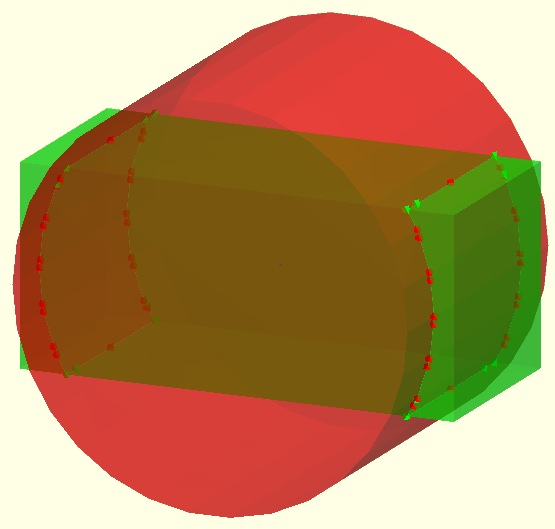% mesh2mesh(facesInA,pointsInA,facesInB,pointsInB) - returns the crossing points of 2 meshes% (by Seven and Thomas, VLFL-Lib, 2015-AUG-07 as class: SURFACES)% % See also: VLFLinpolyhedron, BBiscollofVL, outboundingbox, SGisInterior, % VLcrossingSG, crossingfacets2VLFL% % [trace]=mesh2mesh(facesInA,pointsInA,facesInB,pointsInB)% === INPUT PARAMETERS ===% facesInA: % pointsInA: % facesInB: % pointsInB: % === OUTPUT RESULTS ======% [trace]: %
###### FLfreeboundaryTR4FL(TR4FL)- returns the freeBoundary facets of a tetrahedron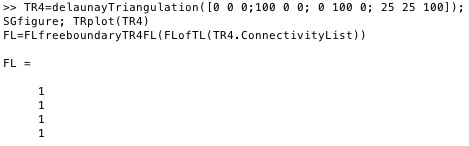% FLfreeboundaryTR4FL(TR4FL) - returns the freeBoundary facets of a % tetrahedron% (by Tim Lueth, VLFL-Lib, 2015-AUG-07 as class: TETRAHEDRONS)% % This procedure is tricky to use. Use only if you complete understand % what you want to do.% % This procedure processes a FL list that contains all surface facets of % a tetrahedron delaunay triangulation (ConnectivityList), created for % instance by % FL=[TL(:,[2 3 4]); TL(:,[2 4 1]); TL(:,[4 3 1]); TL(:,[2 1 3])].% It analyzes which surface has no attached corresponding facet. Those % have to be the outside facets of the tetrahedron delaunay % triangulation, i.e. the freeBoundary.% % There exist in Matlab already a method freeBoundary for a tetrahedron % triangulation (nx4) which returns a facet list. There exist also a % method freeBoundary for a triangle triangulation (nx3) which returns an % edge list.% % (Status of: 2015-08-07)% % fb=FLfreeboundaryTR4FL(TR4FL)% === INPUT PARAMETERS ===% TR4FL: Facet List of a tetrahedron triangulation (FLofTL)% === OUTPUT RESULTS ======% fb: outside, free boundary facet index list% % EXAMPLE: % TR4=delaunayTriangulation([0 0 0;100 0 0; 0 100 0; 25 25 100]); % SGfigure; TRplot(TR4)% FL=FLfreeboundaryTR4FL(FLofTL(TR4.ConnectivityList))%
###### exp_2015_08_07- EXPERIMENT To XOR two solids by delaunay triangulation including crossing points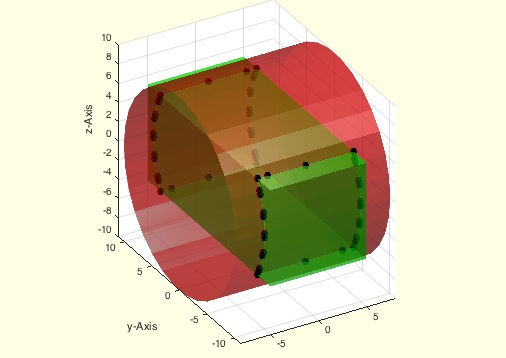%% PUBLISHABLE EXP_2015_08_07 EXPERIMENT TO XOR TWO SOLIDS BY DELAUNAY TRIANGULATION INCLUDING CROSSING POINTS% (by Tim Lueth, VLFL-Lib, 2015-AUG-07 as class: EXPERIMENTS)%%% exp_2015_08_07 - EXPERIMENT To XOR two solids by delaunay triangulation % including crossing points% (by Tim Lueth, VLFL-Lib, 2015-AUG-07 as class: EXPERIMENTS)% % exp_2015_08_07%
###### exp_2015_08_06-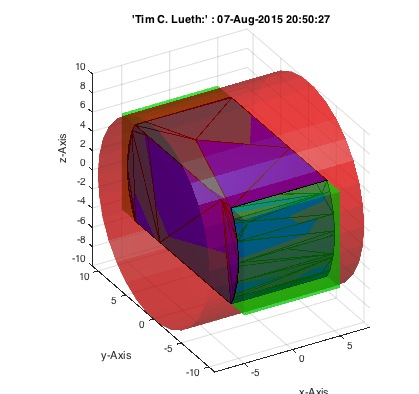% exp_2015_08_06 - % (by Tim Lueth, VLFL-Lib, 2015-AUG-07 as class: EXPERIMENTS)% % exp_2015_08_06%
###### exp_2015_08_05- Experiment for understanding the creation of convex solids from border lines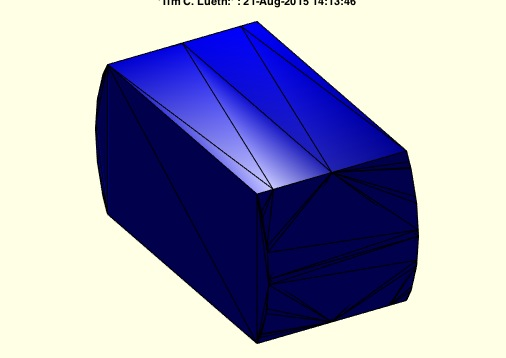%% PUBLISHABLE EXP_2015_08_05 EXPERIMENT FOR UNDERSTANDING THE CREATION OF CONVEX SOLIDS FROM BORDER LINES% (by Tim Lueth, VLFL-Lib, 2015-AUG-21 as class: EXPERIMENTS)%%% exp_2015_08_05 - Experiment for understanding the creation of convex % solids from border lines% % (by Tim Lueth, VLFL-Lib, 2015-AUG-21 as class: EXPERIMENTS)% % [NFL,VL]=exp_2015_08_05% === OUTPUT RESULTS ======% NFL: % VL: %
###### exp_2015_08_04- Experiment to generate tube connectors%% PUBLISHABLE EXP_2015_08_04 EXPERIMENT TO GENERATE TUBE CONNECTORS% (by Tim Lueth, VLFL-Lib, 2015-AUG-05 as class: EXPERIMENTS)%%% exp_2015_08_04 - Experiment to generate tube connectors% (by Tim Lueth, VLFL-Lib, 2015-AUG-05 as class: EXPERIMENTS)% % exp_2015_08_04%
###### FLcontourwallFLn(FLA,FLB,n)- returns a simple wall contour between the boundaries of 2 identical surfaces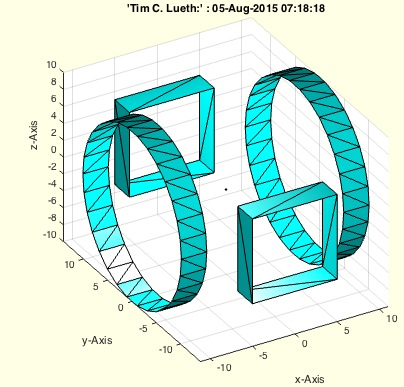% FLcontourwallFLn(FLA,FLB,n) - returns a simple wall contour between the % boundaries of 2 identical surfaces% (by Tim Lueth, VLFL-Lib, 2015-AUG-04 as class: SURFACES)% % FLA and FLB are planar open surfaces. The border line (freeBoundary ist % detected for of them).% % The use of the procedure is shown in exp_2015_08_04 to create tunnels % between the openings of an inner and outer shell. (Status of: % 2015-08-05)% % FLW=FLcontourwallFLn(FLA,FLB,n)% === INPUT PARAMETERS ===% FLA: Facet list describing opening A% FLB: Facet list describing opening B% n: difference number between vertices% === OUTPUT RESULTS ======% FLW: Facet List of the wall contour%
###### SGofVLdelaunay(CVL)- returns a convex delaunay triangulation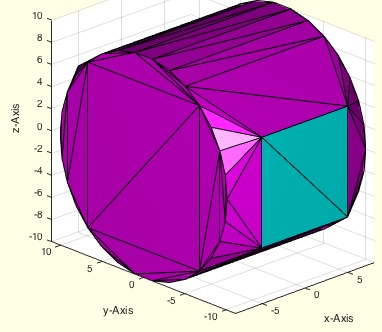% SGofVLdelaunay(CVL) - returns a convex delaunay triangulation% (by Tim Lueth, VLFL-Lib, 2015-AUG-03 as class: SURFACES)% % Based on a cell list of individual vertex list, each is describing the % border/freeBoundary of a planar surface, a delaunay triangulation is % calculated. The tetrahedron triangulation is converted into a surface % triangualtion. A cell list describes the indices of the vertices and % the facet indices within the surface% % At a later stage in makes sense to reorder the facets by [FoL;FiL] % (Status of: 2015-08-04)% % [SG,ViL,FiL,FNL,FoL]=SGofVLdelaunay(CVL)% === INPUT PARAMETERS ===% CVL: Cell list of Contour Vertex Lists {VL1,VL2,VL3} % === OUTPUT RESULTS ======% SG: Solid Geometry (VL=[VL1;VL2,...])% ViL: Vertex index list (n x 2) wit start and end index% FiL: Cells with Facet index Lists% FNL: Facet normal vector list% FoL: Facet index list for the surface without the enclosed ones% % EXAMPLE: A=SGbox([30,10,10]);% B=SGbox([10,30,10]);% [VL,FL,c,d]=SGofVLdelaunay({A.VL(1:end/2,:),A.VL(1+end/2:end,:) % ,B.VL(1:end/2,:),B.VL(1+end/2:end,:)});% VLFLplots(VL,FL,'m');% VLFLplots(VL,FL(d{4},:),'c');% %
###### exp_2015_08_03-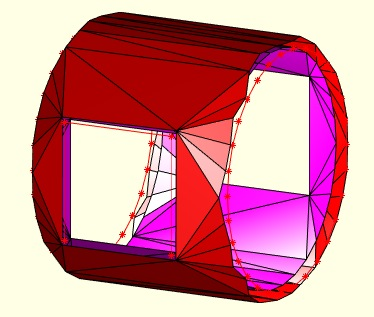% exp_2015_08_03 - % (by Tim Lueth, VLFL-Lib, 2015-AUG-03 as class: EXPERIMENTS)% % exp_2015_08_03%
###### SGgrow(SG,d,thr)- returns the same solid by with increased dimensions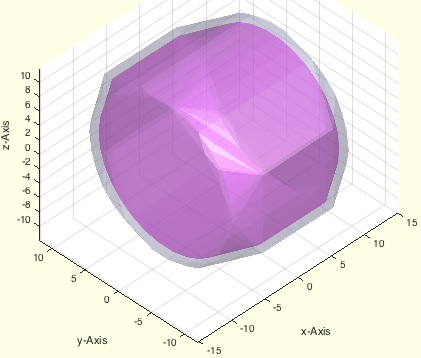% SGgrow(SG,d,thr) - returns the same solid by with increased dimensions% (by Tim Lueth, VLFL-Lib, 2015-AUG-03 as class: SURFACES)% % grows a solid by adding the vertex normal vector to the vertex % coordinates multiplied by a factor d. ATTENTION, The MATLAB-original % vertex normal calculation is not always correct. Therefor in this fnctn % we used a different methods with some side effects too.% SGgrow fnctn is perfect to analyze the correctness of Surface Solids % (Status of: 2017-01-05)% % See also: SGmagnifyVL% % SGN=SGgrow(SG,d,[thr])% === INPUT PARAMETERS ===% SG: Solid Geometry% d: grow distance% thr: threshold for SGselect; default ist 1e-12% === OUTPUT RESULTS ======% SGN: resulting solid geoemtry%
###### SGofBB(BB,d)- returns a solid for a bounding box% SGofBB(BB,d) - returns a solid for a bounding box% (by Tim Lueth, VLFL-Lib, 2015-AUG-03 as class: MODELING PROCEDURES)% % The bounding box can be increased in all direction by an distance % vector. d=[1 1 1] will lead to an enlargement of the bounding box by 2 % mm in the three x y z directions..% For shape optimized cell type bounding boxes use BBofSG directly % (Status of: 2017-04-17)% % See also: BBiscollofVL, BBofVL, CPLofBB, VLFLofBB, outboundingbox, % BBofSG% % SG=SGofBB(BB,[d])% === INPUT PARAMETERS ===% BB: Result of BBofVL, i.e. [xmin xmax ymin ymax zmin zmax]% d: enlargement in [x y z]; default is d=[1 1 1]% === OUTPUT RESULTS ======% SG: Solid box of size of the Bounding Box% % EXAMPLE: % SG=SGofCPLz(PLcircle(10,8),40); SGfigure(SG); view(-30,30);% SGofBB(BBofVL(SG.VL))%
###### TR3ofTR4(TR4,rem)- returns the surface triangulation of a tetrahedron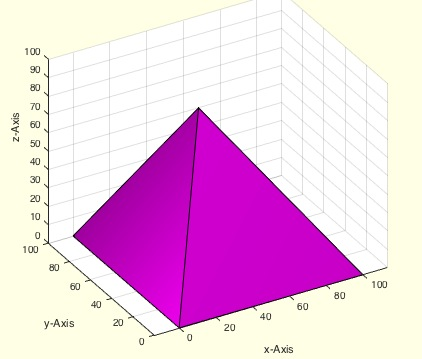% TR3ofTR4(TR4,rem) - returns the surface triangulation of a tetrahedron% (by Tim Lueth, VLFL-Lib, 2015-AUG-02 as class: TETRAHEDRONS)% % Single line procedure:% TR3=triangulation(freeBoundary(TR4),TR4.Points);% The procedure does not (!) remove unnecessary vertices unless rem=true % (default is false) (Status of: 2015-08-07)% % [TR3,VL,FL,SI]=TR3ofTR4(TR4,[rem])% === INPUT PARAMETERS ===% TR4: tetrahedron triangulation% rem: remove unused (internal) vertices; default is false% === OUTPUT RESULTS ======% TR3: surface triangulation% VL: Vertex list% FL: Facet list% SI: FL=SI(FL) => maps to TR4.Points% % EXAMPLE: TR4=delaunayTriangulation([0 0 0;100 0 0; 0 100 0; 25 25 % 100]); % TR3ofTR4(TR4)%
###### exp_2015_08_02- Experiment to generate tube connectors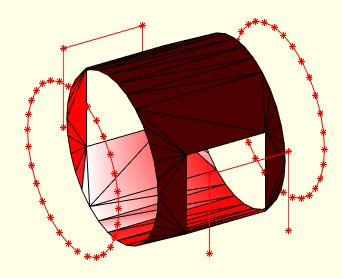%% PUBLISHABLE EXP_2015_08_02 EXPERIMENT TO GENERATE TUBE CONNECTORS% (by Tim Lueth, VLFL-Lib, 2015-AUG-02 as class: EXPERIMENTS)%%% exp_2015_08_02 - Experiment to generate tube connectors% (by Tim Lueth, VLFL-Lib, 2015-AUG-02 as class: EXPERIMENTS)% % exp_2015_08_02%
###### depuseString(sstr)- searches for string using the system command grep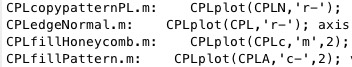% depuseString(sstr) - searches for string using the system command grep% (by Tim Lueth, VLFL-Lib, 2015-AUG-01 as class: FILE HANDLING)% % This fnctn is much faster than depuseTL or depuse, but returns all rows % with the occurrence of the fnctn. It is helpful to decide with the eye % whether older fnctns have to be corrected when changing parameters. % (Status of: 2017-01-04)% % See also: depuseTL, depuseToolbox, publishTL, pcodeTL, depfunTL, % pcodedirTL% % [l,f]=depuseString(sstr)% === INPUT PARAMETERS ===% sstr: search string% === OUTPUT RESULTS ======% l: list of lines in the files of the current dirctory% f: list of mfiles that contains the string% % EXAMPLE: Search for PLplot% depuseString('PLplot')%
###### edgeNormal(VL,VNL,EL)- returns the edge normals of surface boundaries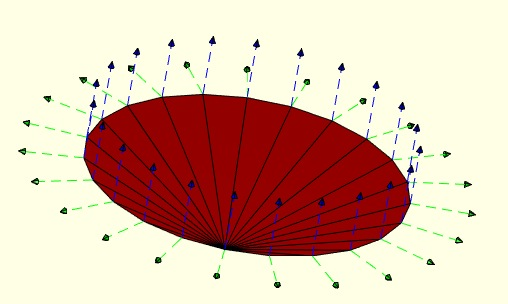% edgeNormal(VL,VNL,EL) - returns the edge normals of surface boundaries% (by Tim Lueth, VLFL-Lib, 2015-AUG-01 as class: SURFACES)% % In case of a planar or freeform surface that is not closed(!) there % exist a border line (freeBoundary). This fnctn calculates the normal % vectors of the edge list vertices, i.e. the cross product of the line % from the previous to the next vertex of a vertex of an edge with the % normal vector of this vertic on the surface. (Status of: 2017-07-09)% % Introduced first in SolidGeometry 2.4% % See also: edgeNormal, CPLedgeNormal, VLedgeNormal, PLnorm, PLELnorm, % PLFLfaceNormal, VLnorm, VLFLnormf, VLFLfaceNormal% % [EOL,c]=edgeNormal(VL,VNL,EL)% === INPUT PARAMETERS ===% VL: Vertex list (nx3)% VNL: Vertex normal list (nx3) (i.e. vertexNormal)% EL: Edge list (i.e. freeBoundary)% === OUTPUT RESULTS ======% EOL: Normal vector of the vertex in the first column of the edge list% c: vertex index list = EL(:,1)% % EXAMPLE: % TR3=triangulation(FL,VL);% NL=vertexNormal(TR3); % EL=freeBoundary(TR3);% [EOL,c]=edgeNormal(VL,NL,EL);% VL0(c,:)=VL(c,:)+s*EOL; % % See also: edgeNormal, CPLedgeNormal, VLedgeNormal, PLnorm, PLELnorm, % PLFLfaceNormal, VLnorm, VLFLnormf, VLFLfaceNormal%
###### ELofjointFL(FL1,FL2)- returns a list of joint edges of two facet list% ELofjointFL(FL1,FL2) - returns a list of joint edges of two facet list% (by Tim Lueth, VLFL-Lib, 2015-JUL-31 as class: SURFACES)% % efficient procedure that uses ismember to detect corresponding edges in % facet lists. Format is:% [start vertex index, end vertex index, FL1 index, FL2 index] % % used if two open sub-surfaces of a solid are linked by an border line % (Status of: 2015-07-31)% % [ELiL]=ELofjointFL(FL1,FL2)% === INPUT PARAMETERS ===% FL1: Facet List 1% FL2: Facet List 2% === OUTPUT RESULTS ======% [ELiL]: Edge list plus Facet Index in FL1 and FL2%
###### separateNaN(VL,i)- separates List by NaN rows entries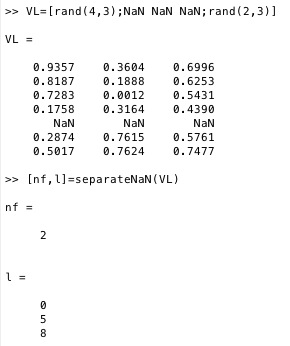% separateNaN(VL,i) - separates List by NaN rows entries% (by Tim Lueth, VLFL-Lib, 2015-JUL-31 as class: AUXILIARY PROCEDURES)% % n=separateNaN(VL); for i=1:n; myfunc(separateNaN(VL,i); end; (Status % of: 2017-06-12)% % See also: selectNaN, replaceNaN, cellofNaN% % x=separateNaN(VL,i)% === INPUT PARAMETERS ===% VL: List of% i: optional number of sublist% === OUTPUT RESULTS ======% x: List i or number of lists if nargin==1% % EXAMPLE: how to use% nf=separateNaN(FL); if nf>1; for i=1:nf; PL=separateNaN(FL,i); end; % Example:% VL=[rand(4,3);NaN NaN NaN;rand(2,3)]% [nf,l]=separateNaN(VL)% separateNaN(VL,2)%
###### SGofSurface(OVL,OFL,t,d,s)- returns a fitting solid relativ to a surface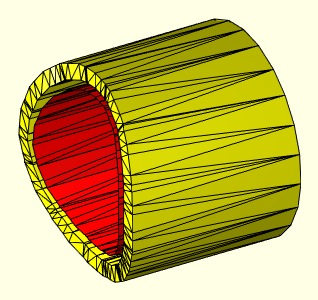% SGofSurface(OVL,OFL,t,d,s) - returns a fitting solid relativ to a surface % (by Tim Lueth, VLFL-Lib, 2015-JUL-30 as class: SURFACES)% % This fnctn supports also facets list of type cell: {FL1,FL2,FL3}.% It also supports facets list separated by NaN: [FL1;NaN NaN NaN;FL2]% IT DOES NOT SUPPORT SURFACES WITH HOLES YET (Status of: 2017-04-17)% % See also: VLtransN% % [SG,VL1,VL2,FLW]=SGofSurface(OVL,OFL,[t,d,s])% === INPUT PARAMETERS ===% OVL: Vertex list of a surface (nx3)% OFL: Facet list of a surface (nx3)% t: thickness of the solid; default is 0.5% d: distance from the surface; default is 0.3% s: streching of the border line; default is 0% === OUTPUT RESULTS ======% SG: resulting solid that can be attached to the surface% VL1: VL oved along d% VL2: VL moved d*ez% FLW: Facet of list wall [VL1;VL2]%
###### exp_2015_07_28- EXPERIMENT to test SGofSurface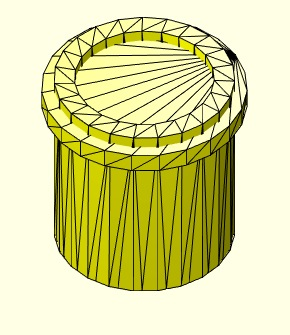% exp_2015_07_28 - EXPERIMENT to test SGofSurface% (by Tim Lueth, VLFL-Lib, 2015-JUL-28 as class: EXPERIMENTS)% % This procedure shows how from surfaces attaching solids can be generated% (Status of: 2015-08-01)% % A=exp_2015_07_28% === OUTPUT RESULTS ======% A: resulting solid body%
###### SGcopyrotZ(SG,rotangz,tdist,n)- copies a solid around the z-axis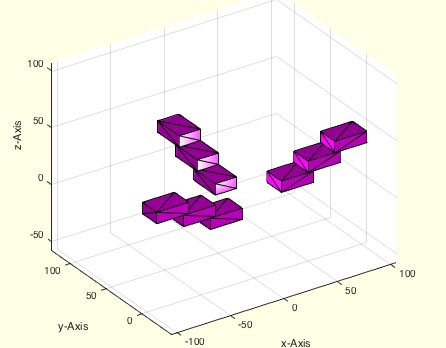% SGcopyrotZ(SG,rotangz,tdist,n) - copies a solid around the z-axis% (by Tim Lueth, VLFL-Lib, 2015-JUL-27 as class: AUXILIARY PROCEDURES)% % See also: SGboxing, SGpatternXYZ, SGpatternRotz, SGarrangeSG, % SGarrangeSGC, SGCaddSGn, SGCaddSG% % SGN=SGcopyrotZ(SG,rotangz,[tdist,n])% === INPUT PARAMETERS ===% SG: Solid geoemtry% rotangz: list of rotation angles% tdist: translation vector before each copy% n: number of repeats% === OUTPUT RESULTS ======% SGN: resulting solid% % EXAMPLE: SGcopyrotZ(SGtrans1(SGbox([30,20,10])),[0 pi/2 pi],[25 0 10],3)% %
###### CPLcopypattern(CPL,number,delta)- creates patterns by copying CPLs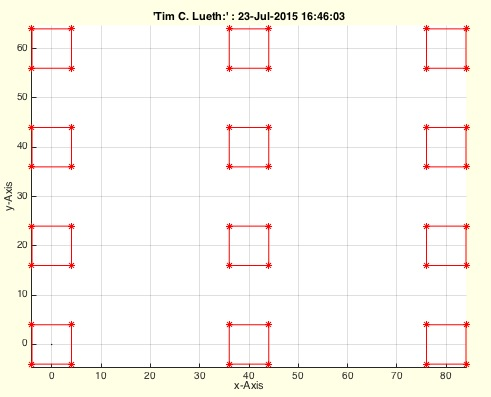% CPLcopypattern(CPL,number,delta) - creates patterns by copying CPLs% (by Tim Lueth, VLFL-Lib, 2015-JUL-23 as class: CLOSED POLYGON LISTS)% % CPLN=CPLcopypattern(CPL,number,delta)% === INPUT PARAMETERS ===% CPL: Original Polygon% number: copy vector [nx ny]% delta: distance vector [dx dy]% === OUTPUT RESULTS ======% CPLN: New closed polygon line%
###### SGpatternXYZ(iSG,n,t)- copies solid geometries along xyz-axis% SGpatternXYZ(iSG,n,t) - copies solid geometries along xyz-axis% (by Laurens De Smedt, VLFL-Lib, 2015-JUL-23 as class: SURFACES)% % See also: SGboxing, SGpatternRotz, SGcopyrotZ, SGarrangeSG, % SGarrangeSGC, SGCaddSGn, SGCaddSG% % oSG=SGpatternXYZ(iSG,n,t)% === INPUT PARAMETERS ===% iSG: original solid geoemtry% n: number of copies wanted% t: degrees(∞) over which the pattern reaches(360∞ if not specified)% === OUTPUT RESULTS ======% oSG: resulting solid geometries%
###### SGpatternRotz(iSG,n,ndeg)- copies solid geometries around the z-axis% SGpatternRotz(iSG,n,ndeg) - copies solid geometries around the z-axis% (by Laurens De Smedt, VLFL-Lib, 2015-JUL-23 as class: AUXILIARY % PROCEDURES)% % See also: SGboxing, SGpatternXYZ, SGcopyrotZ, SGarrangeSG, % SGarrangeSGC, SGCaddSGn, SGCaddSG% % oSG=SGpatternRotz(iSG,n,ndeg)% === INPUT PARAMETERS ===% iSG: original solid geoemtry% n: number of copies wanted% ndeg: degrees(∞) over which the pattern reaches(360∞ if not specified)% === OUTPUT RESULTS ======% oSG: resulting solid geometries%
###### SGparts(Nr,TLPAR,sl,ol)- Often used solid geometries additive designed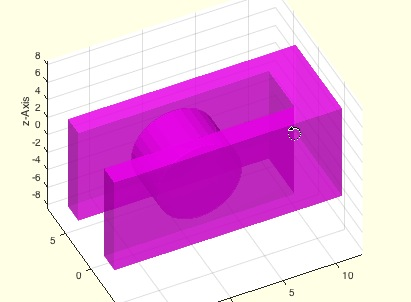% SGparts(Nr,TLPAR,sl,ol) - Often used solid geometries additive designed% (by Tim Lueth, VLFL-Lib, 2015-JUL-16 as class: MODELING PROCEDURES)% % Default parameters are:% Overlap: ol=0.1% Slot distances: sl=0.3% For the rest it is necessary to read the manual% 001 - sz=[x y z] (Box)% 002 - r=radius, h=height (Cylinder)% (Status of: 2015-07-16)% % SG=SGparts(Nr,[TLPAR,sl,ol])% === INPUT PARAMETERS ===% Nr: Part number% TLPAR: Parameterstring for the definition of dimensions% sl: slot size for separated objects; default is 0.5% ol: overlap for additive objects; default is 0.1% === OUTPUT RESULTS ======% SG: Final solid geometry%
###### exp_2015_07_16 (varstr)- EXAMPLE for a very elegant method to transfer variable definitions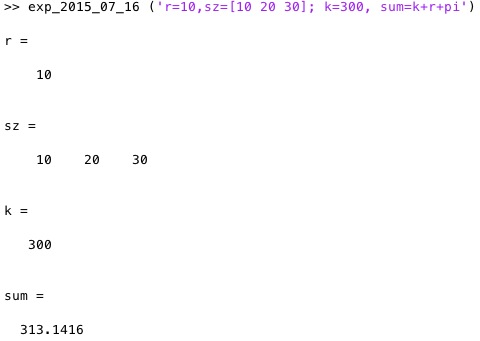% exp_2015_07_16 (varstr) - EXAMPLE for a very elegant method to transfer % variable definitions% (by Tim Lueth, VLFL-Lib, 2015-JUL-16 as class: AUXILIARY PROCEDURES)% % This is not a fast but very elegant method to declare variables by a % parameterstrinf directly in Matlab Syntax (Status of: 2015-07-16)% % exp_2015_07_16(varstr)% === INPUT PARAMETERS ===% varstr: parameter variable string, for example (varargin{1})% % EXAMPLE: exp_2015_07_16 ('r=10,sz=[10 20 30]; k=300, sum=k+r+pi')%
###### evalTLvars (DEFPAR, TLPAR)- modifies variables of a calling function% evalTLvars (DEFPAR, TLPAR) - modifies variables of a calling procedure% (by Tim Lueth, VLFL-Lib, 2015-JUL-16 as class: AUXILIARY PROCEDURES)% % This programming auxiliary procedure creates or modifies existing % variables in the workspace of the calling procedure by evaluating a % string with default parameter values and an optional second string this % is evaulated after the default value string. (Status of: 2015-07-17)% % evalTLvars(DEFPAR,TLPAR)% === INPUT PARAMETERS ===% DEFPAR: Default variable values% TLPAR: Parameter string (varargins) to overload the default values% % EXAMPLE: Create variables by a string: % evalTLvars ('a=12, b=13, c=a+b', varargin{1});%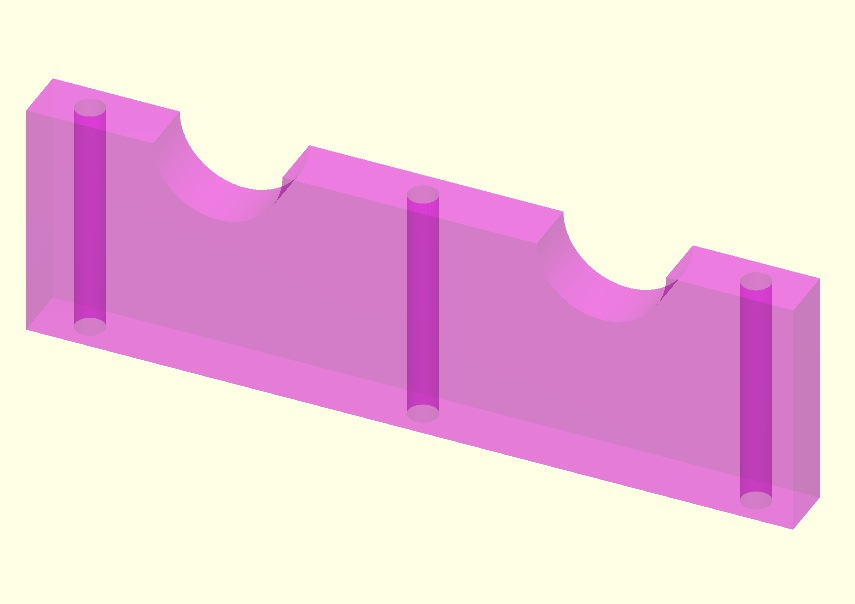% CAD_1_1 - PUBLISHABLE EXAMPLE of Excercise CAD 1.1% (by Tim Lueth, VLFL-Lib, 2015-JUL-14 as class: MODELING PROCEDURES)% % This procedure is an example how to solve the excercise 1.1 of the % "Rechnerintegrierte Produktentwicklung - CAD und CAD/CAM" (2015)% of Professor Dr.-Ing. Udo Lindemann (Status of: 2015-07-14)% % CAD_1_1% % EXAMPLE: web(publish ('CAD_1_1'));%% CAD_1_2 - PUBLISHABLE EXAMPLE of Excercise CAD 1.2% (by Tim Lueth & Laurans de Smedt, VLFL-Lib, 2015-JUN-29 as class: % MODELING PROCEDURES)% % This procedure is an example how to solve the excercise 1.2 of the % "Rechnerintegrierte Produktentwicklung - CAD und CAD/CAM" (2015)% of Professor Dr.-Ing. Udo Lindemann (Status of: 2015-06-29)% % CAD_1_2%
###### SGfigure()- Application for opening and viewing STL Files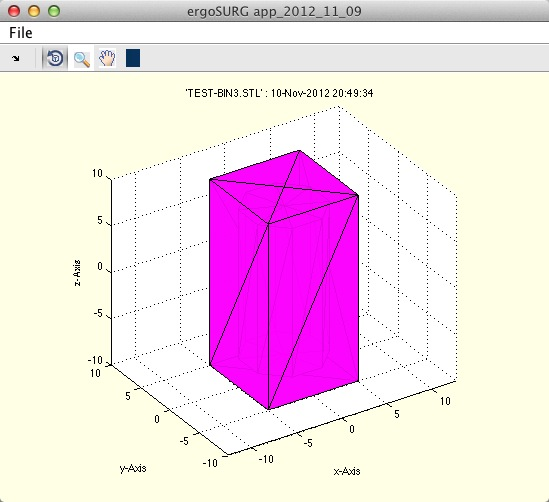% SGfigure() - Application for opening and viewing STL Files% (by Tim Lueth, VLFL-Lib, 2015-JUN-22 as class: VISUALIZATION)% % The procedure can be compiled as application or used as user interface % procedure. Called without input parameters, a file reading dialog will % be opened. There are examples for reading, writing of STL files and % changing light condition or grid information.% It should show the potential of Matlab to the company Arburg, Lossburg % (Status of: 2015-08-26)% % h=SGfigure([])% === OUTPUT RESULTS ======% h: handle to figure% % EXAMPLE: GUI with a small cube (0.1 0.1 0.1) in the center:% SGfigure%
###### PLtoolTip(d1,d2,w)- creates a PL fo SGtoolMilling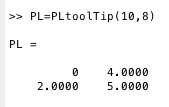% PLtoolTip(d1,d2,w) - creates a PL fo SGtoolMilling% (by Tim Lueth, VLFL-Lib, 2015-JUN-21 as class: MECHANICAL PROCEDURES)% % PL=PLtoolTip(d1,d2,[w])% === INPUT PARAMETERS ===% d1: outer diameter% d2: tip diameter% w: optional angle; default is 90% === OUTPUT RESULTS ======% PL: Point list%
###### SGcut2(SG,z)- Cuts a solid geometry by inserting vertices and facets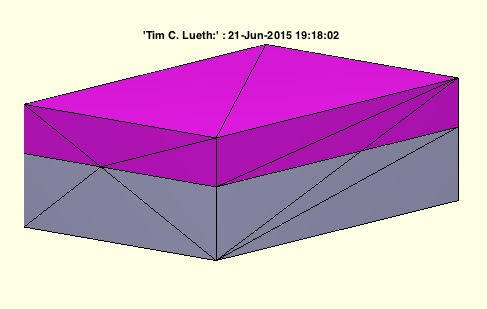% SGcut2(SG,z) - Cuts a solid geometry by inserting vertices and facets% (by Tim Lueth, VLFL-Lib, 2015-JUN-21 as class: SURFACES)% % returns one modified solid that has additional vertices and facets % along the cutting plane. In contrast to SGcut is delivers always % exactly one solid and accepts only one z coordinate. If the fnctns is % called twice with the same z-coordinates, no additional points are % created. For this purpose it was necessary to modify the fnctn % SCslicer. (Status of: 2015-06-21)% % See also: SGcut% % [SGA]=SGcut2(SG,z)% === INPUT PARAMETERS ===% SG: Solid Geometry (VL,FL)% z: z value for slicing% === OUTPUT RESULTS ======% [SGA]: Solid below cutting plane (and above z2)% % EXAMPLE: SGcut2 (SGbox([30,20,10]),1);%
###### exp_2015_06_19- EXPERIMENT that is the origin of SGmilling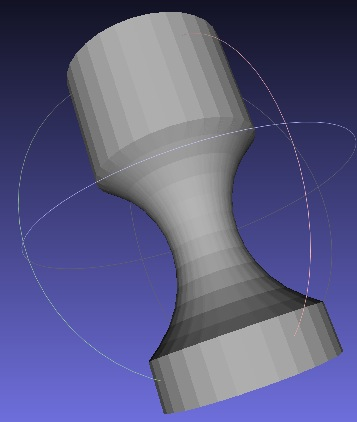% exp_2015_06_19 - EXPERIMENT that is the origin of SGmilling% (by Tim Lueth, VLFL-Lib, 2015-JUN-19 as class: EXPERIMENTS)% % A=exp_2015_06_19% === OUTPUT RESULTS ======% A: % % EXAMPLE: % A=exp_2015_06_19;% VLFLfigure(A)%
###### VILofrangez(VL,FL,z,thr)- returns indices of VL and FL in a cut plane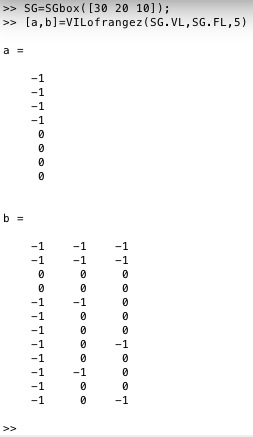% VILofrangez(VL,FL,z,thr) - returns indices of VL and FL in a cut plane% (by Tim Lueth, VLFL-Lib, 2015-JUN-19 as class: SLICES)% % The vertex index list shows relation of the vertex z coordinate related % to the cutting plane: +1 = above plane, -1 under plane, 0=crosses % plane. The crossing facet list explains the crossing situation% 0 0 0 All points in plane (1) % -1 -1 -1 All points under plane (1)% +1 +1 +1 All points above plane (1)% 0 0 +1 One point above (3)% 0 0 -1 One point under (3)% 0 +1 +1 One point on plane, two above (3)% 0 -1 -1 One point on plane, two under (3)% 0 +1 -1 One point on plane, one above, one under (3)% 0 -1 +1 One point on plane, one above, one under (3)% -1 +1 +1 One point under, one point above (3)% +1 -1 -1 One point under, one point above (3)% overall 27 combinatons (Status of: 2015-06-19)% % [VIL,CFL]=VILofrangez(VL,FL,z,[thr])% === INPUT PARAMETERS ===% VL: Vertex list% FL: Facet list% z: cut coordinate% thr: optional grid; default is 1e-5% === OUTPUT RESULTS ======% VIL: +1 = above plane, -1 under plane, 0=crosses plane% CFL: Crossing Facet List% % EXAMPLE: SG=SGbox([30 20 10]);% [a,b]=VILofrangez(SG.VL,SG.FL,5)%
###### SGtoolMilling(A,PL)- returns a solid geometry resulting by a milling process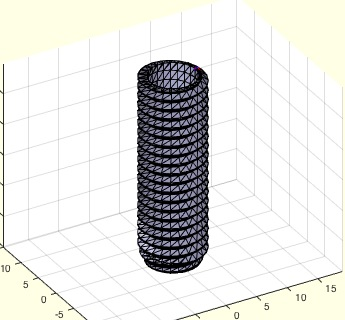% SGtoolMilling(A,PL) - returns a solid geometry resulting by a milling % process% (by Tim Lueth, VLFL-Lib, 2015-JUN-16 as class: SURFACES)% % SG=SGtoolMilling(A,PL)% === INPUT PARAMETERS ===% A: Solid Geometry% PL: rz-List milling contour (nx2) % === OUTPUT RESULTS ======% SG: resulting solid% % EXAMPLE: % A=SGthread(10,30)% SGtoolMilling(A,PLtoolTip(10,8))%
###### SGtoolTip (d1,d2,w)- returns a SG for a "tool" for SGbool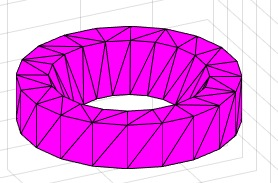% SGtoolTip (d1,d2,w) - returns a SG for a "tool" for SGbool% (by Tim Lueth, VLFL-Lib, 2015-JUN-16 as class: EXPERIMENTS)% % Many DIN and ISO standards for machine elements expect a point tip % shape at the end of a screw. This SGtool can be used as negtive form % for shaping a tip of a bolt or screw etc. (Status of: 2015-06-16)% % SGtoolTip(d1,d2,[w])% === INPUT PARAMETERS ===% d1: outer diameter% d2: tip diameter% w: optional angle; default is 90 degree%
###### exp_2015_06_16 (d1,d2,w)- EXPERIMENT to create a SGBool-Tool for point tips of screws% exp_2015_06_16 (d1,d2,w) - EXPERIMENT to create a SGBool-Tool for point % tips of screws% (by Tim Lueth, VLFL-Lib, 2015-JUN-16 as class: EXPERIMENTS)% % exp_2015_06_16(d1,d2,[w])% === INPUT PARAMETERS ===% d1: outer diameter% d2: tip diameter% w: optional angle; default is 90 degree%
###### SGiso4026(M,L,imb)- SG of Hexagon socket set screw with flat point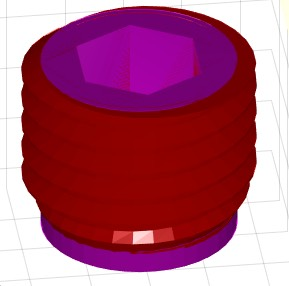% SGiso4026(M,L,imb) - SG of Hexagon socket set screw with flat point % (by Tim Lueth, VLFL-Lib, 2015-JUN-15 as class: MECHANICAL PROCEDURES)% % returns a solid geometry of a "Hexagon socket set screw with flat % point" related to DIN913 or ISO4026 (Status of: 2017-01-29)% % See also: DINhelp, DIN913, DIN912, DIN336, SGiso4762% % SG=SGiso4026(M,L,[imb])% === INPUT PARAMETERS ===% M: Diameter (millimeter)% L: Length (millimeter)% imb: true=imbus; false=simple screw% === OUTPUT RESULTS ======% SG: Solid geoemetry of Hexagon socket set screw with flat point %
###### DIN913(M)- returns the DIN913/ISO4026 table for a metric threads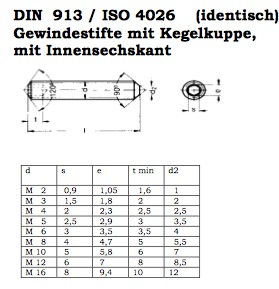% DIN913(M) - returns the DIN913/ISO4026 table for a metric threads% (by Tim Lueth, VLFL-Lib, 2015-JUN-15 as class: MECHANICAL PROCEDURES)% % The table DIN913/ISO4026 has the following columns. All values are % given in millimeter% 1) Metric outer diameter in mm% 2) Imbus width% 3) Tip diameter% 4) unknown% 5) Depth of imbus (Status of: 2017-01-29)% % See also: DINhelp, SGiso4026, DIN912, DIN336, SGiso4762% % [M,TL]=DIN913(M)% === INPUT PARAMETERS ===% M: metric treat diameter% === OUTPUT RESULTS ======% M: M that was used for the table entry% TL: Table entry for M%
###### DINhelp- open different urls for DIN and ISO Standard Machine Elements% DINhelp - open different urls for DIN and ISO Standard Machine Elements% (by Tim Lueth, VLFL-Lib, 2015-JUN-15 as class: MECHANICAL PROCEDURES)% % The German company Wegertseder offeres summaries of DIN/ISO standards % for fixation elements (Status of: 2017-01-29)% % See also: DINhelp, DIN913, SGiso4026, DIN912, DIN336, SGiso4762% % DINhelp% % EXAMPLE: just enter DINhelp for opening the pae%
###### interPL(PL,x)- returns a value as linear interpolation using a PL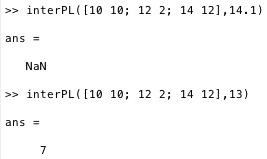% interPL(PL,x) - returns a value as linear interpolation using a PL% (by Tim Lueth, VLFL-Lib, 2015-JUN-15 as class: AUXILIARY PROCEDURES)% % Returns a linear interpolation value y using a Point list (nx2) % consisting of x and y values. If the input parameter is outside of the % defined interval the procedure return NaN. At a later stage it makes % sense to introduce a third parameter for different interpolation % procedures (splines etc.).% ATTENTION: The point list has to be sorted using PL=sortrows(PL,1) % before calling the procedure. (Status of: 2015-06-19)% % y=interPL(PL,x)% === INPUT PARAMETERS ===% PL: Point list (nx2) using x and y values% x: input value within the interval of xmin and xmax of PL% === OUTPUT RESULTS ======% y: interpolated value for y or NaN outside of xmin and xmax of PL% % EXAMPLE: find interpolation values for a simple point list for x=13% interPL([10 10; 12 2; 14 12],13)%